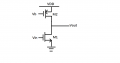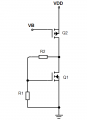# Constant Current Biasing in Microelectronics

#### Kenny Lu

Joined Mar 2, 2018
11
Hi Everyone,
I am a student studying microelectronics at uni this semester and am new to this forum. There is a question about biasing a common source stage using a constant current source that I want to ask.

Please the below for the circuit diagram.

I was using Hspice to simulate my circuit. However, I found that without giving a DC bias voltage to M1, it would remain in Linear despite the fact that M2 was providing a constant current to it. I also found that vds of M1 is extremely low. How does constant current biasing work? Do we still need to provide Vin with a DC component when biasing the circuit using a constant current? Would anyone please help me get this concept clarified?Thank you very muchKenny

#### wayneh

Joined Sep 9, 2010
17,153
I think I know, but can you describe what M1 and M2 are, and how Vin and Vb are defined.

#### Kenny Lu

Joined Mar 2, 2018
11
I think I know, but can you describe what M1 and M2 are, and how Vin and Vb are defined.
Yes absolutely. M1 is an NMOS transistor wired up in a common source topology, whereas M2 is a PMOS transistor functioning as a constant current load. Vin is the signal source and Vb is a DC bias voltage for M2.

Now I can work out the circuit's small-signal gain, which is Av = -gm1 *(ro1//ro2), where the subscript 1 denotes M1 and 2 denotes M2, without a problem.

The question I have is more of a simulation and understanding one. I don't quite understand how constant current biasing works; therefore I don't know what the correct way of simulation should be performed on this circuit using Hspice.

There are a few different ways to bias an amplifier stage and one of them utilises constant current as described in microelectronics. For a Vgs bias, one simply apply a DC bias voltage and the signal to amplified to Vin when simulating the circuit. What about constant current biasing? Should one also apply VGS, the DC bias voltage, to M1?

Thank you very much

Kenny

#### Jony130

Joined Feb 17, 2009
5,241
If both MOSFETs work in a saturation region we have two "current sources" in series. So to properly bias this circuit we almost always using some kind of negative feedback to take care of a DC biasing conditions for M1 and M2.
For example this oneSo, by choosing the R1 and R2 resistors you can set Vout = VDD/2 at quiescent current.
https://electronics.stackexchange.c...d-which-transistor-is-acting-as/336044#336044

#### Kenny Lu

Joined Mar 2, 2018
11
If both MOSFETs work in a saturation region we have two "current sources" in series. So to properly bias this circuit we almost always using some kind of negative feedback to take care of a DC biasing conditions for M1 and M2.
For example this one
View attachment 147503

So, by choosing the R1 and R2 resistors you can set Vout = VDD/2 at quiescent current.
https://electronics.stackexchange.c...d-which-transistor-is-acting-as/336044#336044
Hi Jony130,
Does that mean I need to also give M1 a DC bias voltage when doing Hspice simulation?
(i.e. Vin vin 0 DC 0.6v ...) something of this sort?

Thank you very much
Kenny

#### Jony130

Joined Feb 17, 2009
5,241
You have at least two possibilities.

First - Use R1 and R2 to set the M1 DC bias and add a large capacitor at the input for an AC signal.
But in this case R2 will effect the AC input resistance.

The second one is to remove R1 and R2 from the circuit and just set the proper DC offset at M1 gate in the simulation.

Last edited:

#### Kenny Lu

Joined Mar 2, 2018
11
You have at least two possibilities.

First - Use R1 and R2 to set the M1 DC bias and add a large capacitor at the input for an AC signal.
But in this case R2 will effect the AC input resistance.

The second one is to remove R1 and R2 form the corcuit and just set the proper DC offset at M1 gate in the simulation.
Hi Jony130,
Thank you very much for your help. I appreciate it

Kenny